# pylops.Laplacian¶

pylops.Laplacian(dims, dirs=(0, 1), weights=(1, 1), sampling=(1, 1), edge=False, dtype='float64', kind='centered')[source]

Laplacian.

Apply second-order centered Laplacian operator to a multi-dimensional array.

Note

At least 2 dimensions are required, use pylops.SecondDerivative for 1d arrays.

Parameters: dims : tuple Number of samples for each dimension. dirs : tuple, optional Directions along which laplacian is applied. weights : tuple, optional Weight to apply to each direction (real laplacian operator if weights=[1,1]) sampling : tuple, optional Sampling steps for each direction edge : bool, optional Use reduced order derivative at edges (True) or ignore them (False) for centered derivative dtype : str, optional Type of elements in input array. kind : str, optional Derivative kind (forward, centered, or backward) l2op : pylops.LinearOperator Laplacian linear operator ValueError If dirs. weights, and sampling do not have the same size

Notes

The Laplacian operator applies a second derivative along two directions of a multi-dimensional array.

For simplicity, given a two dimensional array, the Laplacian is:

$y[i, j] = (x[i+1, j] + x[i-1, j] + x[i, j-1] +x[i, j+1] - 4x[i, j]) / (\Delta x \Delta y)$

## Examples using pylops.Laplacian¶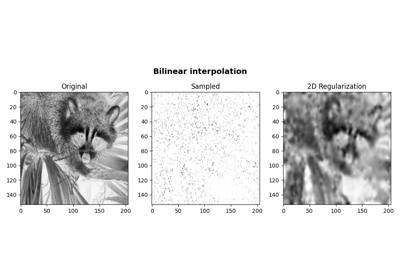Bilinear Interpolation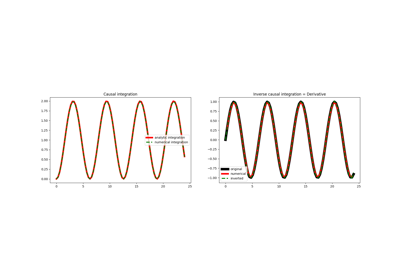Causal Integration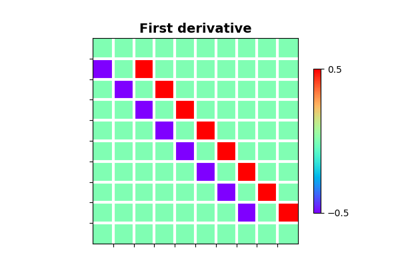Derivatives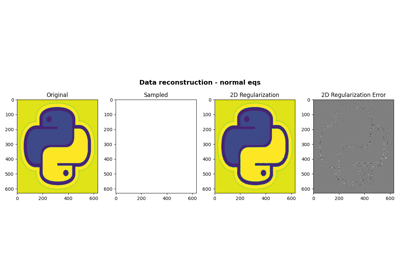06. 2D Interpolation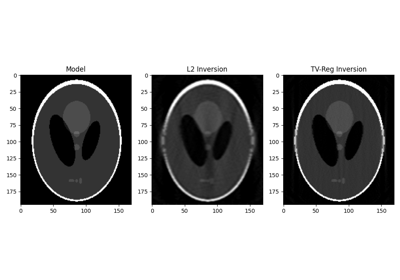16. CT Scan Imaging